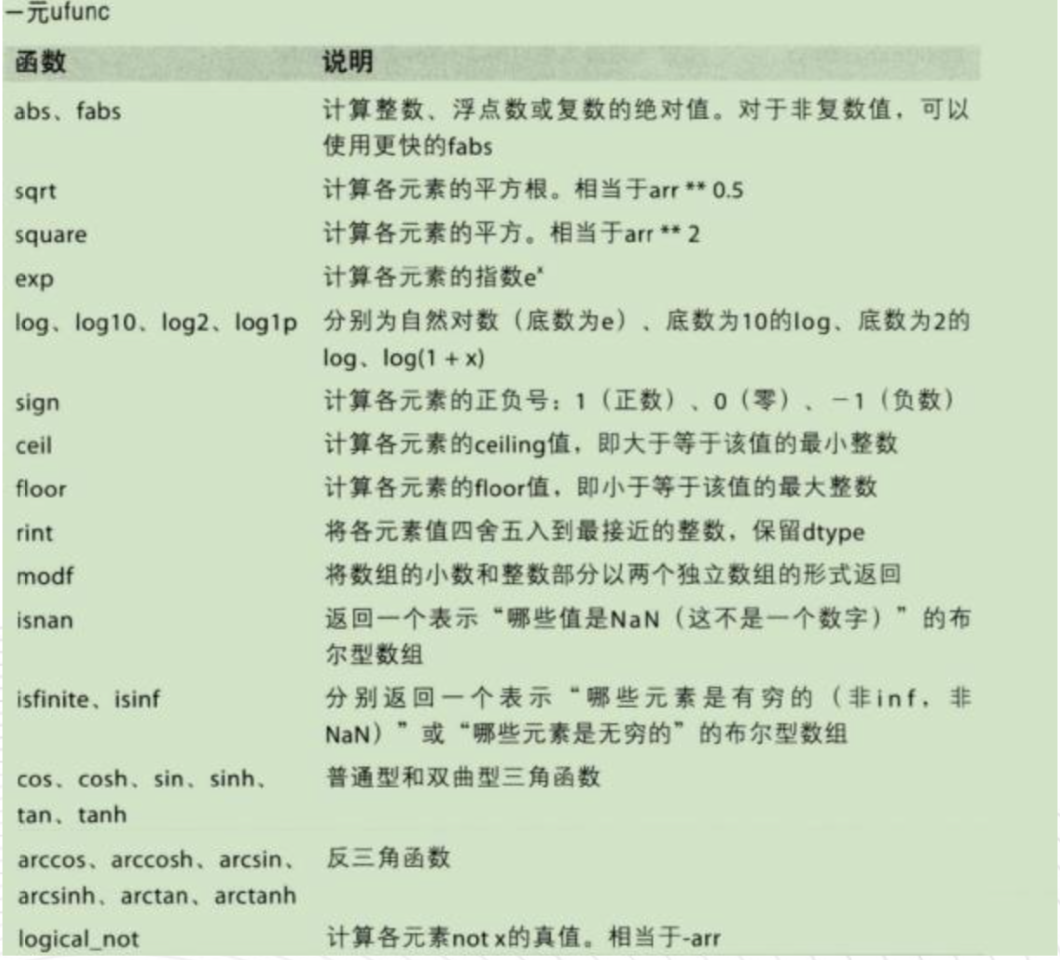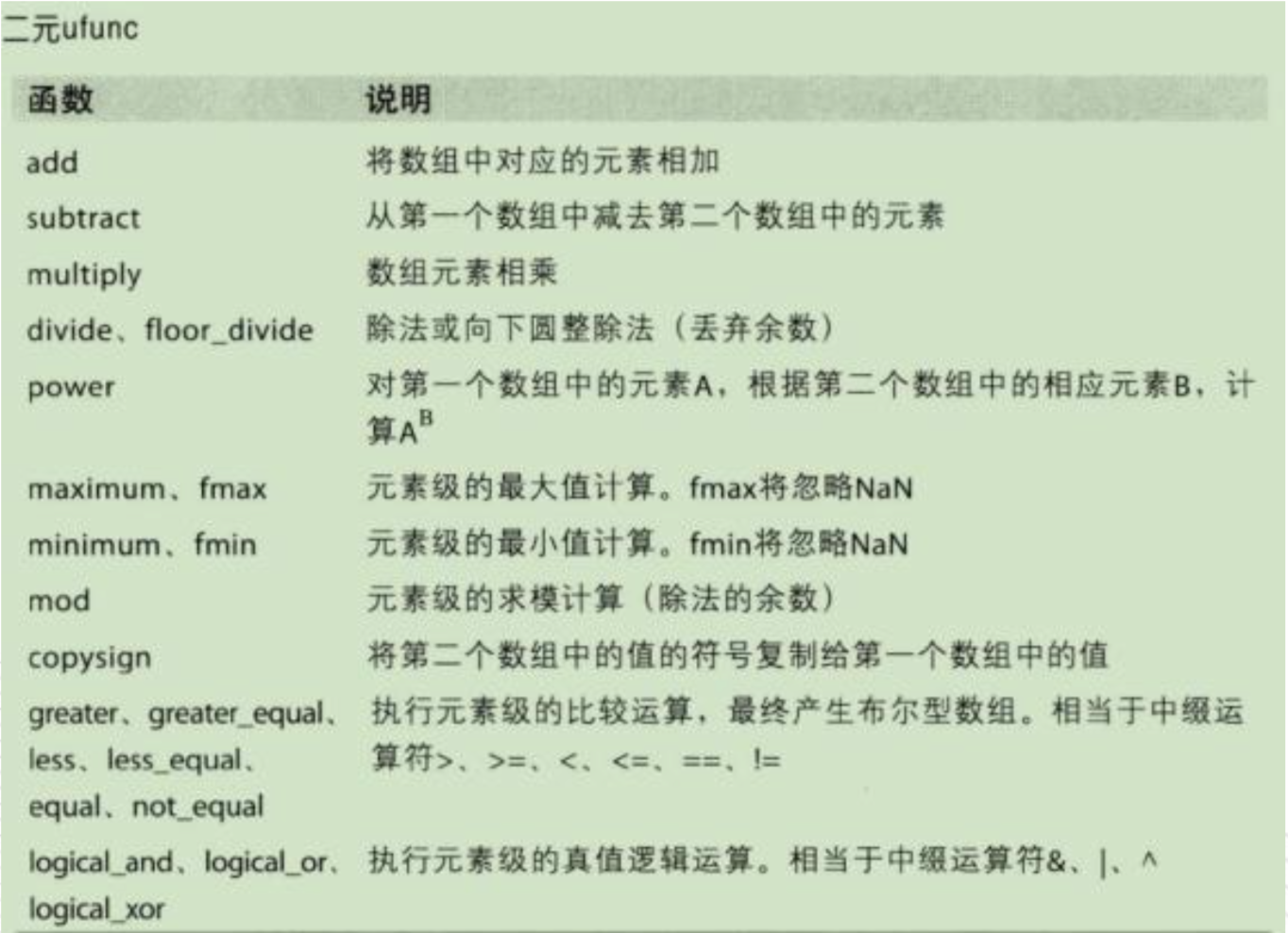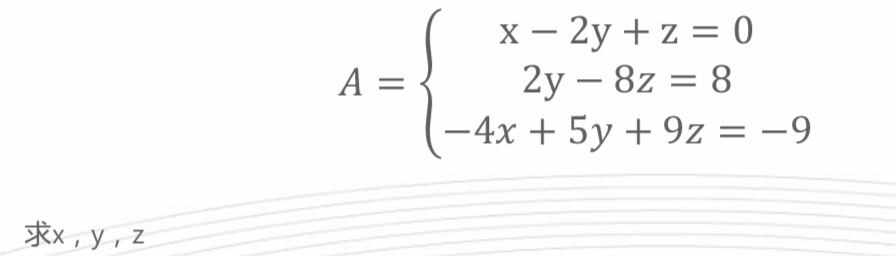• 因为自己有线性代数的基础，所以吴老师讲解的一些线性代数的基础知识就不一一列举，这里只归纳部分知识。 1.案例1 通过矩阵的运算，我们可以快速计算出多个房价的预测值，极大加快速度，如下图： 2.逆矩阵 逆矩阵：...
因为自己有线性代数的基础，所以吴老师讲解的一些线性代数的基础知识就不一一列举，这里只归纳部分知识。
1.案例1
通过矩阵的运算，我们可以快速计算出多个房价的预测值，极大加快速度，如下图：2.逆矩阵
逆矩阵：如果矩阵A是一个m*m的方阵，且可逆，则不存在逆矩阵的矩阵称为非奇异矩阵或退化矩阵。


展开全文吴恩达 机器学习
• 线性代数高清中文版，本书是一本 优 秀 的现代教材，给出新的线性代数基本介绍和一些有趣应用，目的是帮助学生掌握线性代数的基本概念及应用技巧，为后续课程的学习和工作实践奠定基础。与以前的版本相比，第4版增加...
• 因为工作原因，重新复习线性代数，现做《线性代数及其应用》笔记，以备个人整理复习所用。 第一章 线性代数中的线性方程组 定理1（简化阶梯型矩阵的唯一性）P13 每个矩阵行等价于唯一的简化阶梯型矩阵 定理2...
因为工作原因，重新复习线性代数，现做《线性代数及其应用》笔记，以备个人整理及复习所用。

第一章 线性代数中的线性方程组

定理1（简化阶梯型矩阵的唯一性）P13

每个矩阵行等价于唯一的简化阶梯型矩阵

定理2（存在于唯一性定理）P19

线性方程组相容的充要条件是增广矩阵的最右列不是主元列。

若方程组相容，则没有自由变量，有唯一解。有自由变量，有无穷多解。

定理3 P35

向量方程和矩阵方程等价。

定理4 P36

重要的定理，见原书

定理5 P38

矩阵和向量的运算规则

定理6 P45

定理7 （线性相关集特征）P57

定理8 P58

1.1 线性方程组

从线性方程组开始，讨论线性方程组的解的问题，有如下3种情况：

1. 无解（不相容）

2. 唯一解（相容）

3. 无穷多解（相容）

然后引入高斯消元法来解线性方程组。

1.2 行化简与阶梯型矩阵

行化简，基本变量和自由变量。引入定理2.

1.3 向量方程

从向量角度来看

1.4 矩阵方程

从向量角度，到矩阵角度

1.5 线性方程组的解集

平凡解（过零），非平凡解。如题，从特解扩展到整个解集。

1.6 线性方程组的应用

1.7 线性无关

引入了线性无关和线性相关概念

1.8 线性变换

引入线性变换的概念，不陌生

1.9 线性变化矩阵

这个概念很熟悉，唯一有用的就是本节介绍的方法对于记忆线性变换矩阵很有作用

总结

1. 无解（不相容），不是每一行都有主元

2. 唯一解（相容），每一行都有主元，没有自由变量，变换矩阵A可逆，行列式不为0

3. 无穷多解（相容），每一行都有主元，有自由变量

这样，主元、自由变量、行列式、可逆（对于方阵）这些特性就串联起来了。


展开全文• 本书是一本优秀的现代教材，给出最新的线性代数基本介绍和一些有趣应用，目的是帮助学生掌握线性代数的基本概念及应用技巧，为后续课程的学习和工作实践奠定基础。与以前的版本相比，第3版中的概念更加形象化，而且...
• 线性代数及其应用(原书第3版)》是一本优秀的现代教材，给出最新的线性代数基本介绍和一些有趣应用，目的是帮助学生掌握线性代数的基本概念及应用技巧，为后续课程的学习和工作实践奠定基础。与以前的版本相比，第3...
• 本书是一本优秀的现代教材，给出新的线性代数基本介绍和一些有趣应用，目的是帮助学生掌握线性代数的基本概念及应用技巧，为后续课程的学习和工作实践奠定基础。与以前的版本相比，第4版增加了两张内容——几何向量...
• 本书包括6章内容： 行列式及其应用、矩阵、线性方程组与向量、矩阵的特征值与特征向量、二次型Mathematica软件应用·每章都配有习题，书末给出了习题答案·本书在编写中力求重点突出、由浅入深、 通俗易懂·本书可...
• 文章目录对称矩阵与二次型1 实对称矩阵的对角化-谱分解1.1 含义1.2 实对称矩阵的特征向量是正交的1.3 分解条件1.4 分解方法1.5 谱分解的其他意义1.6 谱分解的应用2. 复数矩阵与酉矩阵2.1 复数向量2.1.1 复数向量的模...
对称矩阵与二次型
文章目录对称矩阵与二次型1 实对称矩阵的对角化-谱分解1.1 含义1.2 实对称矩阵的特征向量是正交的1.3 分解条件1.4 分解方法1.5 谱分解的其他意义1.6 谱分解的应用2. 复数矩阵与酉矩阵2.1 复数向量2.1.1 复数向量的模长2.1.2 复数向量的内积2.1.3 复数向量的正交2.2 复数矩阵2.2.1 复数矩阵的对称2.2.1 复正交矩阵--酉矩阵3. 二次型3.1 二次型的定义3.2 二次型系数与矩阵的对应关系3.2.1 二次型系数与矩阵A的关系3.2.2 二次型系数与矩阵A的最简形的关系3.3 主轴定理（同谱分解的应用)3.3.1 基于变量代换的二次型简化3.3.2 二次型矩阵A的特征值和特征向量的含义3.3.3 主轴定理的几何意义3.4 二次型的分类3.5 正定矩阵3.5.1 正定矩阵的定义3.5.2 正定矩阵的判定方法3.5.3 正定矩阵与最小二乘4. 相似矩阵5 奇异值分解5.1 奇异值分解的含义5.2 奇异值分解的条件5.3 奇异值分解的方法5.3.1 法15.3.2 法25.4 长方形矩阵的奇异值分解5.4.1 m>n型5.4.2 n>n型5.5 奇异值分解深度剖析5.6 奇异值分解的应用5.6.1 误差估算5.6.2 分解得四个子空间的基向量5.6.3 估计A的秩6. 主成分分析6.1 含义6.2 步骤6.2.1数据中心化6.2.2 直线拟合6.2.3 用拉格朗日乘数法解向量方向v6.2.4 用特征值评定各个主成分的价值量高低
1 实对称矩阵的对角化-谱分解
1.1 含义
谱分解又叫做对称矩阵的对角化，也就是说，必须是能进行对角化的对称矩阵，才能进行谱分解。
1.2 实对称矩阵的特征向量是正交的
$根据对角化 A = S*λ*S^{-1}$
因为A是对称矩阵，有A=A^T
$A^T = (S^{-1})^T*λ^T*S^T=S*λ*S^{-1}$
所以有S-1=ST
可知S必定是一个标准正交阵，原对角分解可化为
$A = Q*∑*Q^T$
1.3 分解条件
谱分解的条件是矩阵A必须是能进行对角化的对称矩阵
1.4 分解方法
分解方法与对角化是一样的，都是先求特征值，再求特征向量，最后求得矩阵Q和∑。因为Q是标准正交阵，最后不需要求逆，只要转置就好，计算量更加的小
1.5 谱分解的其他意义
如果把谱分解式子乘开，可得
$A = λ1*v1*v1^T+....+λn*vn*vn^T$
因为vi都是标准正交基，所以A可以看做是vi的投影矩阵的线性组合
1.6 谱分解的应用
谱分解的主要应用在坐标变换上，能够把二次型曲线通过谱分解变换到标准位置，比如椭圆，可以把长轴和短轴变换到坐标轴上来，能够使得计算更加方便。在二次型中，得到的特征向量方向就是二次型的极值存在的方向，比如椭圆的长轴和短轴方向。而特征值标准二次型的极值，比如椭圆的长轴和短轴大小。
下面介绍如何通过谱分解，把二次型变换到标准位置。假设二次型为X^T*A*X
首先通过变量代换，令Y = p*X，p是标准正交基矩阵，也就是A的特征向量矩阵，X = pT*Y可得
$X^T*A*X = Y^T*P*A*P^T*Y = Y^T*∑*Y$
我们看出，通过谱分解代换，可以将原来的二次型变换成了一个没有交叉相乘项的二次型，也就是特征值矩阵。
2. 复数矩阵与酉矩阵
2.1 复数向量
2.1.1 复数向量的模长
$||Z||=\bar{z}^T*z$
2.1.2 复数向量的内积
$(\bar{x})^T*y$
2.1.3 复数向量的正交
$(\bar{qi})^T*qj$
2.2 复数矩阵
2.2.1 复数矩阵的对称
$\bar{A}^T=A$
2.2.1 复正交矩阵–酉矩阵
$\bar{Q}^T*Q=I$
3. 二次型
3.1 二次型的定义
假设有未知变量xi组成的向量
$X = \left\{\begin{matrix}x_1 &...& x_n\end{matrix}\right\}$
A是一个对称矩阵，二次型
$Q(X)=X^T*A*X$
其方程形式为
$c_1*x_1^2+....+c_n*x_n^2+d1*x_1*x_2+...+d_m*x_i*x_j$
如果A是一个2x2的矩阵的话，对应的二次型不外乎椭圆和双曲线两种，如果是nxn矩阵的话，对应的二次型包括碗面（正定或负定的二次型）、抛物面（半正定或者半负定的二次型）、马鞍面（不定的二次型）等
3.2 二次型系数与矩阵的对应关系
3.2.1 二次型系数与矩阵A的关系
因为A是对称矩阵，所以二次型系数和矩阵A的元素之间存在以下关系：对称矩阵A的对角线位置(i,i)放置的是xi^2的系数。其余对称位置(i,j)放置的是xi*xj的系数的一半
$假设Q(x)=ax1^2+bx2^2+cx3^2+d*x1x2+e*x1x3+f*x2x3$
$则 A = \left\{\begin{matrix}a &d/2& e/2\\d/2&b&f/2\\e/2&f/2&c\end{matrix}\right\}$
3.2.2 二次型系数与矩阵A的最简形的关系
主元位置放置的是配方以后平方项的系数
3.3 主轴定理（同谱分解的应用)
3.3.1 基于变量代换的二次型简化
下面介绍如何通过谱分解，把二次型变换到标准位置。假设二次型为XT*A*X
首先通过变量代换，令Y = p*X，p是标准正交基矩阵，也就是A的特征向量矩阵，X = pT*Y可得
$X^T*A*X = Y^T*P*A*P^T*Y = Y^T*∑*Y$
我们看出，通过谱分解代换，可以将原来的二次型变换成了一个没有交叉相乘项的二次型，也就是特征值矩阵。
3.3.2 二次型矩阵A的特征值和特征向量的含义
在二次型中，得到的特征向量方向就是二次型的极值存在的方向，比如椭圆的长轴和短轴方向。而特征值标准二次型的极值，比如椭圆的长轴和短轴大小。
3.3.3 主轴定理的几何意义
主轴定理将任一二次图形变换到标准位置。
3.4 二次型的分类

正定的：特征值全部大于0
负定的：特征值全部小于0
不定的：特征值有大于0的，也有小于0的
半正定的：特征值大于等于0

3.5 正定矩阵
3.5.1 正定矩阵的定义
如果对称矩阵的特征值全部大于0，那么就称这个矩阵叫做正定矩阵
3.5.2 正定矩阵的判定方法

正定矩阵的二次型恒大于0
所有特征值都是正数
所有主元都是正数
所有子行列式都是正数

3.5.3 正定矩阵与最小二乘
$A^T*A必定是正定矩阵$
在最小二乘部分有证明，此处略
4. 相似矩阵
相似具有具有相同的特征值，但是一般不具有相同的特征向量，如果说A和B相似，则二者有如下关系
$A = S^{-1}*B*S$
举例–特征值的矩阵
$λ = S^{-1}*A*S$
所以A的特征值矩阵和A其实是相似的
5 奇异值分解
5.1 奇异值分解的含义
奇异值分解来源于如下的向量变换，将空间行向量v变换为列空间向量u，其中σ叫做奇异值
$A*V = σ*u$
矩阵形式为
$A = U*∑*V^T$
奇异值分解实际上就是将矩阵A分解为两个正交矩阵U、V和一个对角阵∑的过程。如果A是mxn的，那么U是mxm的，∑是mxn的，V是nxn的
5.2 奇异值分解的条件
任何矩阵都可以做奇异值分解
5.3 奇异值分解的方法
5.3.1 法1
第一种方法是分别求AT*A和A*A^T的特征向量，因为
$A^T*A = V*(∑)^2*V^T$
$A*A^T = U*(∑)^2*U^T$
同时可知A的奇异值就是AT*A的特征值开根号
5.3.2 法2
第二种方法是利用定义求U
$A^T*A = V*(∑)^2*V^T$
上式可以求得特征向量v和奇异值σ
$A*v = σ*u$
$u = \frac{A*V}{||σ||}$
5.4 长方形矩阵的奇异值分解
5.4.1 m>n型
m>n型的矩阵是细长的，因为左奇异矩阵U必须是m*m的，但是特征值至多有n个，其余不足的应该利用正交性质 u1*u2 = 0，也就是解uT*x=0,从左零空间里面获得基向量，同时注意应该做施密特正交化得到标准正交向量
5.4.2 n>n型
n>m型矩阵是矮胖的，因为右奇异矩阵V必须是n*n的，而特征值至多有m个，不足以获得足够的v向量，其余的应该利用正交特征，利用 A*X = 0，从零空间里面补充基向量，同时也应该做施密特正交化变成标准正交矩阵
5.5 奇异值分解深度剖析
这里来分析一下v向量和u向量的组成成分
$A*v = u$
通过上式，我们知道，A的重新线性组合必然也还在其列空间内，所以，向量u应该属于A的列空间，而其余一部分u必须与u1等向量正交，与列空间正交的向量位于左零空间中，所以左奇异矩阵的向量来源为列空间和左零空间，正好列空间与左零空间基向量的和为m
$A*v_{r+1}=0$
因为并不是所有的奇异值都是非零值，当大于标号r以后的奇异值都是零值。所以，我们得到了上面的式子，可以说明，向量v的一部分成分为A的零空间。而另外一部分v与向量vr+1等正交，零空间的正交向量位于行空间中，所以v实际上来源为零空间和行空间的基向量，正好零空间和行空间的基向量和为n
5.6 奇异值分解的应用
5.6.1 误差估算
如果最大的奇异值和最小的奇异值差距过大，那么有微小误差引入会使得系统有较大的误差
5.6.2 分解得四个子空间的基向量
5.6.3 估计A的秩
A的秩基本上就是非零奇异值的个数
6. 主成分分析
6.1 含义
主成分分析主要用于数据的降维
6.2 步骤
6.2.1数据中心化
$\bar{X} = ∑\frac{(x_i)}{n}$
$\hat{x_i} = x_i - \bar{X}$
6.2.2 直线拟合
将数据中心化以后，我们需要在这组数据沿着那条直线分散最大，也就是方程越大，因为数据分散度比较大，也就意味着能够提供的信息量越大。我们假设选定一个方向向量v，让所有数据点在方向向量上投影，投影量的方差作为评价依据，方差越大，分散度越大
我们求数据在某个方向上的方差，则
$Q(x)=∑\frac{(xi*v-\bar{X}*v)^2}{n-1}$
也就是xi在方向v上的投影-平均在v方向上的投影，求投影量的方差，因为做过了数据中心化，均值为0，则有
$Q(x)=∑\frac{(xi*v)^2}{n-1}=v^T*∑\frac{xi^T*xi}{n-1}*v=v^T*\frac{X^T*X}{n-1}*v$
$其中令∑=∑\frac{xi^T*xi}{n-1}，∑数据的协方差$
6.2.3 用拉格朗日乘数法解向量方向v
约束条件为方向向量的模为1
$L = v^T*∑*v+λ(1-v^T*v)$
L对v的导数为0，可得
$∑*v=λ*v$
所以最大分散方向，其实就是协方差矩阵的特征向量，这些方向叫做主成分
6.2.4 用特征值评定各个主成分的价值量高低
特征值占所有特征值的和比例越大，含有有效信息的比例越高。如果一些主成分特征值的比例和高于百分之九十几了，剩余的主成分可以抛弃不要了，就起到了数据降维的作用。


展开全文• 其实书名应该叫线性代数及应用，经典的教程，不比MIT的GILBERT STRONG写的线性代数导论差，两本都看当然更好
• 2020/2/4;2020/2/4;2020/2/4;2020/2/4;2020/2/4;2020/2/4;2020/2/4;2020/2/4;2020/2/4;2020/2/4;2020/2/4;2020/2/4;2020/2/4;2020/2/4;2020/2/4;2020/2/4;2020/2/4;2020/2/4;2020/2/4;2020/2/4;...20
• 二、线性代数应用 numpy.linalg 模块包含线性代数的函数。使用这个模块，可以计算逆矩阵、求特征值、解线性方程组以及求解行列式等。 计算逆矩阵 使用inv 函数计算逆矩阵: import numpy as np A = ...

一、矩阵的创建和算术运算

矩阵的创建：
1、手动创建: mat(‘字符串’)
字符串格式：行与行之间用分号隔开；行内的元素之间用空格隔开。
A=np.mat('1 2 3;4 5 6; 7 8 9')

2、使用Numpy数组创建
1）使用mat(ndarray)函数创建矩阵(利用reshape函数)
B=np.mat(np.arange(1,10).reshape(3, 3))

2）利用mat(ndarray)函数创建矩阵，矩阵中存放随机数(numpy.random)
# 产生随机正态分布样本
C = np.mat(np.random.normal(size=(4,4)))
# 产生2行3列均匀分布随机数组
D = np.mat(np.random.rand(2,3))
# 三行三列正态分布随机数据
E = np.mat(np.random.randn(3,3))
# (1,100)以内的5行5列随机整数
F = np.mat(np.random.randint(1,100,[5,5]))
# (0,1)以内10个随机浮点数
G = np.mat(np.random.random(10))
# [0,10)内随机选择一个数
H = np.random.choice(10)

3）从已有矩阵创建新矩阵：利用bmat()函数创建矩阵(np.eye)

先创建2个小矩阵
再使用bmat函数复合

import numpy as np
A = np.eye(2)
B = 2 * A
C = np.bmat('A B;A B;B A')

算术运算
add  # 将数组中对应的元素相加
subtract   # 从第一个数组中剪去第二个数组中的元素
multiply  # 数组元素相乘
divide、floor_divide  # 除法或向下取整除法（丢弃余数）

1）矩阵的除法运算

divide函数:做除法，整除
true_divide函数:返回除法的浮点数结果
floor_divide函数:做除法，进行向下取整并返回整数  使用/运算符相当于调用divide函数
运算符//对应于floor_divide函数

2）模运算

remainder函数:逐个返回两个数组中元素相除后的余数
mod函数与remainder函数的功能完全一致
%操作符仅仅是remainder函数的简写
fmod函数所得余数的正负由被除数决定，与除数的正负无关

通用函数
1） 概念：通用函数(ufunc)是一种对ndarray中的数据执行元素级运算的函
数。你可以将其看作简单函数(接受一个或多个标量值，并产生一个或多 过标量值)的矢量化包装器。通用函数的输入是一组标量，输出也是一组 标量，它们通常可以对应于基本数学运算，如加、减、乘、除等。
2） 类别：
通用函数(ufunc)有两种类别:
(1)一元(unary)ufunc，它们接受一个数组。返回一个结果数组，当然也 能返回两个数组(modf函数)，但是这种的不是很常见;(2)二元(binary)ufunc，它们接受两个数组，并返回一个结果数组。(3)  创建通用函数
import numpy as np
# 定义一个函数
def ultimate_answer(a):
result = np.zeros_like(a)  # 使用zeros_like函数创建一个和a形状相同， 并且元素全部为0的数组result
result.flat = 42  # .flat 设置数组元素的值为42
return result

# 使用 frompyfunc 创建通用函数
# 创建通用函数，1个输入，1个输出
ufunc = np.frompyfunc(ultimate_answer,1,1)

(4) numpy已有的通用函数的方法

accumulate
reduceat
outer
reduce

# reduce计算，功能是:等价于对矩阵元素求和.
a = np.arange(10)
np.add.reduce(a)

# accumulate计算，功能是：将存储运算的中间结果并返回
np.add.accumulate(a)

# reduceat计算，需要输入一个数组以及一个索引值列表作为参数
np.add.reduceat(a,[0,5,2,7])
#结果为:【10 5 20 15】 #第一步用到索引值列表中的0和5，对数组中索引值在0到5之间的元素进行reduce操作 得到10 #第二步用到索引值5和2。由于2比5小，所以直接返回索引值为5的元素 得到5 #第三步用到索引值2和7。对索引值在2到7之间的数组元素进行reduce操作 得到20 #第四步用到索引值7。对索引值从7开始直到数组末端的元素进行reduce操作 得到15

# outer计算:返回一个矩阵，将第一个矩阵的第一个元素与第二个 矩阵的每个元素求和返回一个矩阵，再将第一个矩阵的第二个元素与第 二个矩阵的每个元素求和返回一个矩阵
np.add.outer(np.add.outer(np.arange(1,4),a))



二、线性代数应用

numpy.linalg 模块包含线性代数的函数。使用这个模块，可以计算逆矩阵、求特征值、解线性方程组以及求解行列式等。
计算逆矩阵
使用inv 函数计算逆矩阵:
import numpy as np
A = np.mat('0 1 2; 1 0 3;4 -3 8')
inverse = np.linalg.inv(A)

解线性方程组
使用solve函数计算线性方程组import numpy as np
A = np.mat('1 -2 1;0 2 -8;-4 5 9')
b = np.array([0,8,-9])
C = np.linalg.solve(A,b)
print('x=',C,'y=',C,'z=',C)

特征值和特征向量
特征值(eigenvalue)即方程 Ax = ax 的根，是一个标量。其中，A 是一 个二维矩阵，x 是一个一维向量。特征向量(eigenvector)是关于特征值的 向量。在numpy.linalg模块中，eigvals函数可以计算矩阵的特征值，而 eig函数可以返回一个包含特征值和对应的特征向量的元组。
import numpy as np
A = np.mat('3 -2;1 0')
eigenvalues, eigenvectors = np.linalg.eig(A)
print('特征值',eigenvalues,'特征向量',eigenvectors)

奇异值分解
SVD(Singular Value Decomposition，奇异值分解)是一种因 子分解运算，将一个矩阵分解为3个矩阵的乘积。在numpy.linalg模块 中的svd函数可以对矩阵进行奇异值分解。该函数返回3个矩阵——U、 Sigma和V，其中U和V是正交矩阵，Sigma包含输入矩阵的奇异值。(np.diag)
import numpy as np
A = np.mat('4 11 14;8 7 -2')
U,Sigma,V = np.linalg.svd(A,full_matrices=False)

计算矩阵的行列式
对于一个n×n的实数矩阵，行列式描述的是一个线性变换对“有向 体积”所造成的影响。行列式的值为正表示保持了空间的定向(顺时针 或逆时针)，为负则表示颠倒了空间的定向。numpy.linalg模块中的 det函数可以计算矩阵的行列式。
import numpy as np
A = np.mat('3 4;5 6')
np.linalg.det(A)


三、专用函数

排序函数
1、ndarray类的sort方法——可对数组进行原地排序;
语法格式: list.sort([axis])
2、argsort函数——返回输入数组排序后的下标;
语法格式: np.argsort(a[, axis, kind, order])
3、sort函数——返回排序后的数组;
语法格式: np.sort(a[, axis, kind, order])
搜索函数

argmax函数——返回数组中最大值对应的下标
语法格式: np.argmax(a)
nanargmax函数——与argmax提供相同的功能，但忽略NaN值
语法格式: np.nanargmax(a)
argmin函数——返回数组中最小值对应的下标
语法格式: np.argmin(a)
nanargmin函数——与argmin的功能类似，但忽略NaN值
语法格式: np.nanargmin(a)
argwhere函数——根据条件搜索非零的元素，并分组返回对应的下标
语法格式: np.argwhere(条件)
searchsorted函数——为指定的插入值寻找维持数组排序的索引位置。该函数使用二分 搜索算法
语法格式: np. searchsorted(a,b)

数组元素抽取

使用nonzero函数抽取数组中的非零元素
使用extract函数基于生成的条件从数组中抽取元素

四、在金融方面的应用

fv函数——计算所谓的终值(future value)，即基于一些假设给出的某个金融资 产在未来某一时间点的价值。

终值由以下4个参数决定——利率、期数、每期支付金额以及现值

语法格式如下: numpy.fv(rate, nper, pmt, pv[, when='end’])

#参数:
rate:每一期的利率(rate of interest)。
nper:期数。
pmt:payment。每期支付金额
pv:present value，现值。
when:{{‘begin’, 1}, {‘end’, 0}}, {string, int}, optional. 每一期的开头还是结尾付


pv函数——计算现值(present value)，即金融资产当前的价值。

现值有以下4个参数决定——利率、期数、每期支付金额以及终值
语法格式如下: numpy.fv(rate, nper, pmt, fv[, when='end’])
#参数:
rate:每一期的利率(rate of interest)。
nper:期数。
pmt:payment。每期支付金额
fv: future value，终值。
when:{{‘begin’, 1}, {‘end’, 0}}, {string, int}, optional. 每一期的开头还是结尾付


npv函数——计算净现值(net present value)，即按折现率计算的净现金流之和。

净现值是指投资方案所产生的【现金净流量】(流入-流出)以资金成本为贴现
率折现之后与原始投资额现值的差额经济意义

NPV>0表示项目实施后，除保证可实现预定的收益率外，尚可获得更高的收益。
NPV<0表示项目实施后，未能达到预定的收益率水平，而不能确定项目已亏损。
NPV=0表示项目实施后的投资收益率正好达到预期，而不是投资项目盈亏平衡。

语法格式如下: numpy.npv(rate, values)
#参数:
rate:折现率。
values: 现金流，正数代表‘收入’或‘取款’，负数代表‘投资’或‘存款’。 第一个值 必须是初始的投资，也就是必须是负数


pmt函数——根据本金和利率计算每期需支付的金额。

语法格式如下: numpy.pmt(rate, nper, pv[, fv=0, when='end’])
#参数:
rate:每一期的利率(rate of interest)
nper:期数
pv:present value，现值


nper函数——计算定期付款的期数。

语法格式如下: numpy.nper(rate, pmt, pv, fv=0, when='end')
#参数:
rate:每一期的利率(rate of interest)
pmt:每期支付的金额 pv:present value，现值
fv: 终值


rate函数——计算利率(rate of interest)。

语法格式如下: numpy.rate(nper, pmt, pv, fv)
#参数:
nper :需还的期数
pmt:每期支付的金额
pv:present value，现值
fv: 终值



展开全文矩阵运算...